# Determine surge and swab pressure for close-ended pipe

I would like to show more calculation about surge and swab pressure. In this article, I will show how to use the previous formulas (Surge and swab pressure method 1) to figure out surge and swab pressure for close-ended pipe (plug flow). The calculation concept is pretty much the same but I would like to show you how much difference between surge/swab if you have the close-ended pipe.

I still use the same data but this time so I can compare apple to apple with the previous calculation.

Example

The well information is listed below:

θ300 = 85

θ600 = 130

Hole diameter = 9 inch

Drill Collar = 6.25 inch

ID of drill collar = 2.5 inch

Drillpipe diameter = 5 inch

ID of drill pipe = 3.5 inch

Average pipe running or pulling speed = 250 ft/min

Drill Pipe Length = 12000 ft

Drill Collar Length = 800 ft

Current Mud Weight = 12.5 ppg

Well TVD = 9000 ft

2nd case – closed ended pipe

Determine pressure loss around drill pipe

1. Determine n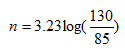n= 0.613

2. Determine K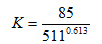K=1.863

3. Determine fluid velocity around drill pipe

For closed ended pipe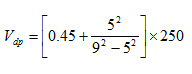Vdp = 224.1 ft/min

4. Maximum pipe velocity

Vm = 224.1 x 1.5 = 336 ft/min

5. Pressure loss around drill pipe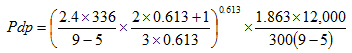Pdp =540.7 psi

Determine pressure loss around drill collar

We still use the same n and K.

1. Determine fluid velocity around drill collar

For open ended pipe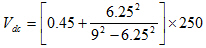Vdc = 345.4 ft/min

2. Maximum pipe velocity

Vm = 345.4 x 1.5 = 518 ft/min

3. Pressure loss around drill collar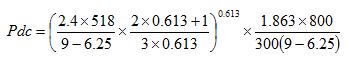Pdc = 86 psi

Total pressure loss = Pdp + Pdc

Total pressure loss = 540.7 + 86 = 626.7 psi

Determine surge pressure with the following equation.Surge pressure = hydrostatic pressure + total pressure loss

Surge pressure = (0.052 x 9000 x 12.5) + 626.7 = 6,476.6 psi

Determine swab pressure with the following equation.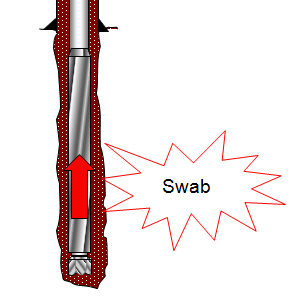Swab pressure = hydrostatic pressure – total pressure loss

Swab pressure = (0.052 x 9000 x 12.5) – 626.7 = 5,223.3 psi

When compared with 1st case (surge and swab pressure for open-ended pipe), you can see that the closed ended pipe creates more surge and swab effect.

Share the joy
Tagged , , , . Bookmark the permalink.